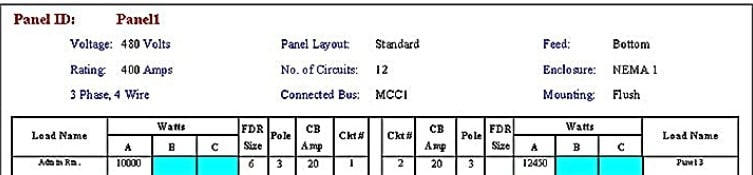# Unbalanced Load Flow Study and AnalysisCarelabs is authorized provider of Electrical Installation’s Study, Analysis, Inspection, and Certification services in UAE, and offer unbalanced load flow study and analysis services.

Load flow analysis is an important function for power system planning and practical studies. Certain applications, particularly in distribution automation and optimization of a power system, require repeated load flow solution and in these applications, it is very important to solve the load flow problem as efficiently as possible.

As the power distribution networks become more complex, there is greater demand for efficient and reliable system operation. Consequently, the most important system analysis tool, load flow studies, should be capable of handling various system configurations with adequate accuracy and speed.

In several situations, it observed that the radial distribution systems remain unbalanced due to single-phase, two-phase and three-phase loads. Thus, load flow solution for unbalanced case, special analysis is necessary.

Usual load flow method cannot directly apply to distribution systems. The mode used for three phase power flow analysis in unbalanced systems cannot develop by extending the single phase balanced methods. A three-phase load flow method has to analyze problems like modeling of different forms of fundamental connections that would regulate starting point for three phase power flow solution as there are phase shifts and transformation ratios for each phase and at different buses. For un-transposed lines and cables the balanced models are no longer useful.

The symmetrical component transformation can decouple the three phases. For three phase networks burden matrix obtain. A method to measure power losses in unbalanced radial distribution systems.

## How to Model the Transformer for Power Flow Analysis.

### Fast Decoupled Power Flow Method for Unbalanced Radial Distribution Systems

This method prefers the laterals instead of buses into layers, it would decrease the problem size to the number of laterals. Using lateral variable rather than bus variable makes this methods adequate for given system topology, however it may add some obstacle if the network topology changed frequently, which is common in distribution systems because of switching operations. Finding three-phase radial distribution networks. This method uses the forward and backward propagation to measure branch currents and bus voltages.

The three-phase Current Injection Method, injection equations written in rectangular regulates full Newton method. Also it shows quadratic convergence properties and convergence obtained for all except some conditioned cases. This uses G-matrix for power flow based on equal current injections. The Network Topology which uses two matrices, viz. Bus Injection to Branch Current and Branch-Current to Bus-Voltage matrices, to find out the solution, modeling of transformer and other components of distribution systems, load flow solution of unbalanced radical distribution networks.

Improved load flow method for unbalanced Radical Distribution System using sequence components. The different connections of transformer in as unbalanced radial distribution networks. Three phase load flow method by solving algebraic recursive expression of voltage significance.

## Load Flow Solution of Unbalanced Radial Distribution Networks

Efficient method for load flow analysis plays an important role in automation algorithms of Radical Distribution System whose scope encloses fault isolation, network reconfiguration and service restoration. Automation algorithm is capable of handling these complex tasks that need frequent topological variations in the Radical Distribution System which demands a dynamic topology processor based on a well-defined data structure.

### Unbalanced Radial Distribution System

Unbalanced radial distribution system can be modeled as a network of buses connected by distribution lines, switches or transformers. Each bus may have a corresponding load, shunt capacitor and co-generator connected to it. This model represented by a radial interconnection of copies of the usual building block for the analysis of power transmission line, two fundamental assumptions made, namely:

### Three-phase Currents Balanced

Transposition of the conductors to get balanced line parameters. However, distribution systems do not give themselves to either of the two assumptions. Due to dominance of single-phase loads, the assumptions of balanced three-phase currents are not applicable.

Distribution lines are hardly changed, nor can it be assumed that the conductor configuration is an equilateral triangle. When two assumptions are invalid, it is necessary to introduce another proper method of calculating the line obstruction.

In distribution systems loads exist in one, two and three phase loads with delta connections. Also loads can differentiated into four types depending on the load characteristics like constant power, constant impedance, constant current and complex loads. In this chapter, the loads considered as constant power type and can mathematically represented as follows

At times the primary feeder supplies loads through distribution transformers tapped at various locations along line section. If each load point modeled as a bus topology, there will be large number of bus topology in the system. Hence these loads represented as lumped loads:

• At one-fourth length of line from sending a dummy bus created at which two third of the load assumed to connect.
• The remaining one-third load assumed to connect at the receiving end bus.

### Transformer

The impact of the transformers in a distribution system is significant. Transformers affect system loss, <span”>zero sequence current, method of grounding and protection strategy.

### Core Loss

The core loss of a transformer approximated by shunt core loss functions on each phase of the secondary terminal of the transformer. These core loss approximation functions based on the results of load modeling analysis which analyze that real and reactive power loss in the transformer core can express as functions of the terminal voltage of the transformer. Transformer core loss functions represented in per unit at the system power base.